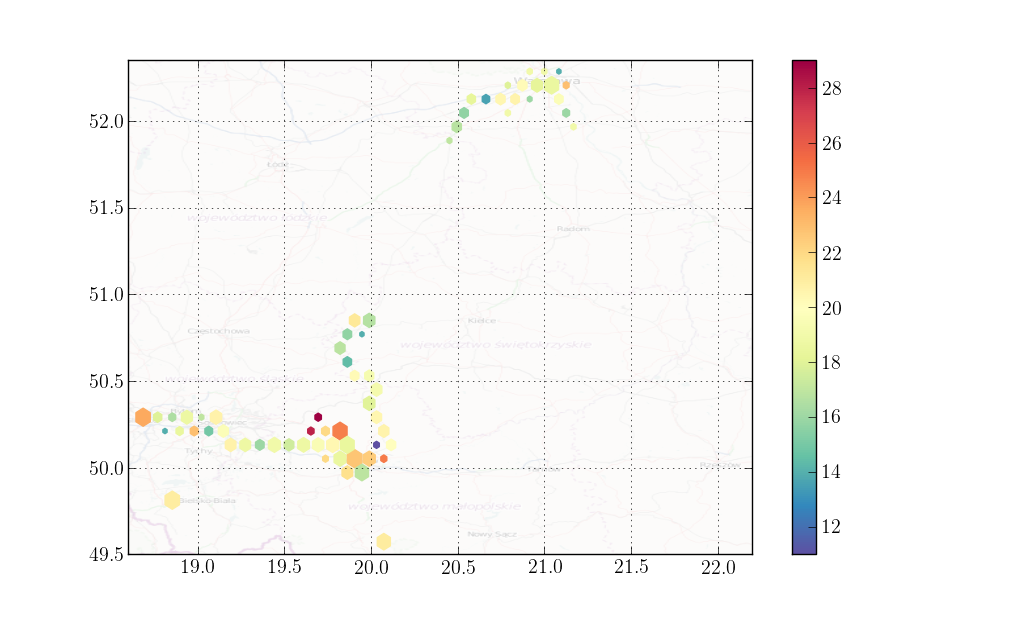# Modifying matplotlib's hexbin to scale hexes

When showing radiation data with matplotlib's hexplot I wanted to somehow include also statistical uncertainties. I didn't see any solution with standard functionality but I came up with a simple modification to make hexes with large uncertainties smaller.Actual scaling of the hexes is done by these few lines (polygons in matplotlib are just arrays of vertices):

``````# scale all polygons
new_polygons = []
for vs, cnts in zip(polygons, accum_hexscale):
xs, ys = vs.T
mx = mean(xs)
my = mean(ys)

sc = hexscale(cnts)

xs = (xs - mx) * sc + mx
ys = (ys - my) * sc + my
new_polygons.append(zip(xs, ys))

polygons = new_polygons
``````

My modification can actually scale hexes with an arbitrary function of bin counts. And if you provide `C` parameter `reduce_C_function` can be separately set for scaling. If you want to just scale hexes according to counts in each bin you can use `arctan` to restrict scaling to [0,1):

``````from pylab import *
from hexbin2 import hexbin2
x = randn(9000)
y = randn(9000) / 2
hexbin2(x, y, hexscale=lambda x: arctan(x/5) * 2. / pi)
``````

Which produces a plot like this:For radiation data I want the hex color to indicate an average radiation in bin's area so `reduce_C_function` is set to `mean`. On the other hand, uncertainty of measurement is square root of total counts in bin's area, so `hexbin_reduce_C_function` is set to `sum`.

Full code prepared as a separate module: https://gist.github.com/anonymous/5278510